# Python语音识别

• 首先 ，语音识别允许机器捕捉我们说的单词，短语和句子
• 第二 ，自然语言处理，让机器了解我们所说的话，和
• 第三 ，语音合成让机器说话。

## 构建语音识别器

### 开发语音识别系统的困难

• 词汇量的 大小 - 词汇量的 大小会影响ASR的开发。考虑以下大小的词汇表以便更好地理解。
• 例如，在语音菜单系统中，小型词汇表由2-100个单词组成
• 例如，在数据库检索任务中，中等大小的词汇表由几个100到1,000个单词组成
• 大型词汇表由几万个单词组成，如一般的听写任务。

• 渠道特征 - 渠道质量也是一个重要方面。 例如，人类语音包含具有全频率范围的高带宽，而电话语音包括具有有限频率范围的低带宽。请注意，后者更难。
• 说话模式 - 易于开发ASR还取决于说话模式，即语音是处于隔离字模式，连接字模式还是连续语音模式。 请注意，连续语音难以识别。
• 口语风格 - 朗读演讲可以采用正式风格，也可以采用休闲风格自发和对话。 后者更难以识别。
• 说话者依赖 - 语音可以是说话者相关的，说话者自适应的或说话者独立的 独立的扬声器是最难建立的。
• 噪声类型 - 噪声是开发ASR时需要考虑的另一个因素。 信噪比可能在不同的范围内，具体取决于观察较少的声学环境与较多的背景噪声 -
• 如果信噪比大于30dB，则认为是高范围
• 如果信噪比介于30dB到10db之间，则认为是中等SNR
• 如果信噪比小于10dB，则认为是低范围

• 麦克风特性 - 麦克风 的质量可能很好，平均或低于平均水平。此外，嘴和微型电话之间的距离可能会有所不同。识别系统也应考虑这些因素。

## 可视化音频信号 - 从文件读取并处理它

### 例

import numpy as np
import matplotlib.pyplot as plt
from scipy.io import wavfile


frequency_sampling, audio_signal = wavfile.read("/Users/admin/audio_file.wav")


print('\nSignal shape:', audio_signal.shape)
print('Signal Datatype:', audio_signal.dtype)
print('Signal duration:', round(audio_signal.shape /
float(frequency_sampling), 2), 'seconds')


audio_signal = audio_signal / np.power(2, 15)


audio_signal = audio_signal [:100]
time_axis = 1000 * np.arange(0, len(signal), 1) / float(frequency_sampling)


plt.plot(time_axis, signal, color='blue')
plt.xlabel('Time (milliseconds)')
plt.ylabel('Amplitude')
plt.title('Input audio signal')
plt.show()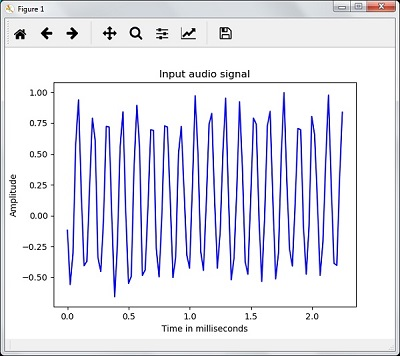Signal shape: (132300,)
Signal Datatype: int16
Signal duration: 3.0 seconds


## 表征音频信号：转换为频域

### 范例

import numpy as np
import matplotlib.pyplot as plt
from scipy.io import wavfile


frequency_sampling, audio_signal = wavfile.read("/Users/admin/sample.wav")

print('\nSignal shape:', audio_signal.shape)
print('Signal Datatype:', audio_signal.dtype)
print('Signal duration:', round(audio_signal.shape /
float(frequency_sampling), 2), 'seconds')


audio_signal = audio_signal / np.power(2, 15)


length_signal = len(audio_signal)
half_length = np.ceil((length_signal + 1) / 2.0).astype(np.int)


signal_frequency = np.fft.fft(audio_signal)


signal_frequency = abs(signal_frequency[0:half_length]) / length_signal
signal_frequency **= 2


len_fts = len(signal_frequency)


if length_signal % 2:
signal_frequency[1:len_fts] *= 2
else:
signal_frequency[1:len_fts-1] *= 2


signal_power = 10 * np.log10(signal_frequency)


x_axis = np.arange(0, len_half, 1) * (frequency_sampling / length_signal) / 1000.0


plt.figure()
plt.plot(x_axis, signal_power, color='black')
plt.xlabel('Frequency (kHz)')
plt.ylabel('Signal power (dB)')
plt.show()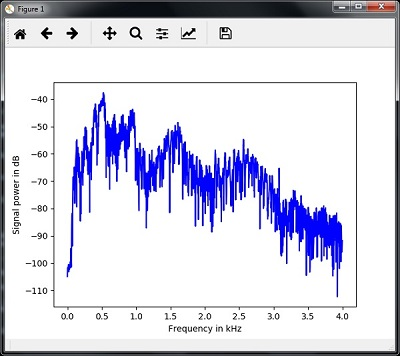## 生成单调音频信号

### 范例

import numpy as np
import matplotlib.pyplot as plt
from scipy.io.wavfile import write


output_file = 'audio_signal_generated.wav'


duration = 4 # in seconds
frequency_sampling = 44100 # in Hz
frequency_tone = 784
min_val = -4 * np.pi
max_val = 4 * np.pi


t = np.linspace(min_val, max_val, duration * frequency_sampling)
audio_signal = np.sin(2 * np.pi * tone_freq * t)


write(output_file, frequency_sampling, signal_scaled)


audio_signal = audio_signal[:100]
time_axis = 1000 * np.arange(0, len(signal), 1) / float(sampling_freq)


plt.plot(time_axis, signal, color='blue')
plt.xlabel('Time in milliseconds')
plt.ylabel('Amplitude')
plt.title('Generated audio signal')
plt.show()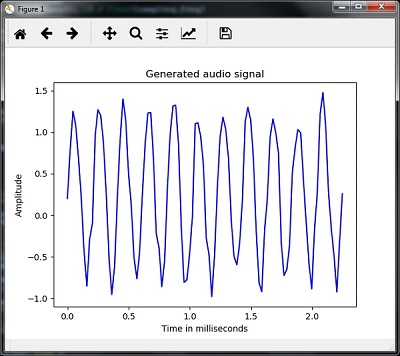## 语音特征提取

### 范例

import numpy as np
import matplotlib.pyplot as plt
from scipy.io import wavfile
from python_speech_features import mfcc, logfbank


frequency_sampling, audio_signal = wavfile.read("/Users/admin/audio_file.wav")


audio_signal = audio_signal[:15000]


features_mfcc = mfcc(audio_signal, frequency_sampling)


print('\nMFCC:\nNumber of windows =', features_mfcc.shape)
print('Length of each feature =', features_mfcc.shape)


features_mfcc = features_mfcc.T
plt.matshow(features_mfcc)
plt.title('MFCC')


filterbank_features = logfbank(audio_signal, frequency_sampling)


print('\nFilter bank:\nNumber of windows =', filterbank_features.shape)
print('Length of each feature =', filterbank_features.shape)


filterbank_features = filterbank_features.T
plt.matshow(filterbank_features)
plt.title('Filter bank')
plt.show()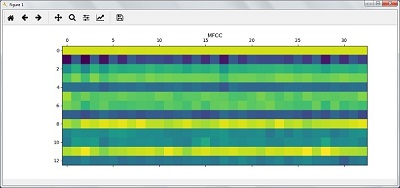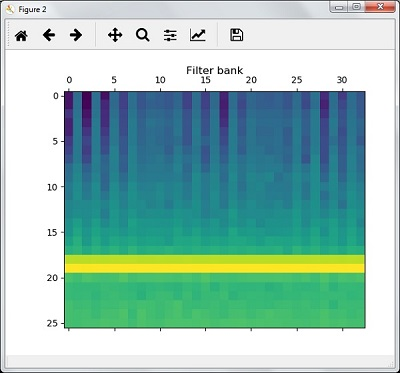## 对口语的认识

• Pyaudio - 可以使用 pip install Pyaudio 命令 安装
• SpeechRecognition - 可以使用 pip install SpeechRecognition 安装此软件包

### 范例

import speech_recognition as sr


recording = sr.Recognizer()


with sr.Microphone() as source: recording.adjust_for_ambient_noise(source)
audio = recording.listen(source)


try:
except Exception as e:
print(e)


Please Say Something:
You said:


codingdict.com


Python启发式搜索：启发式搜索在人工智能中起着关键作用。AI中的启发式搜索的概念启发式是一个经验法则，它引导我们找到可能的解决方案。人工智能中的大多数问题具有指数性质并且具有许多可能的解决方案。您不确切知道哪些解决方案是正确的，并且检查 ...